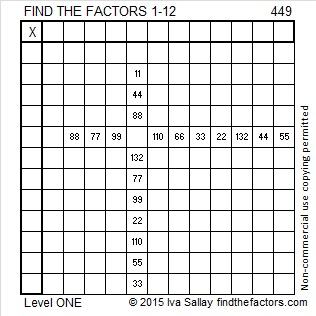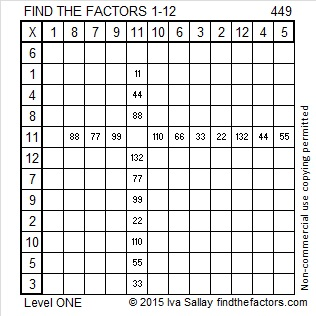# 449 and Level 1

449 is the sum of consecutive prime numbers. How many and what are they? Check the comments to see the answer.Print the puzzles or type the factors on this excel file:  12 Factors 2015-04-06

449 = 20² + 7² so 449 is the hypotenuse of a Pythagorean triple. That triple will be 2(20)(7), 20² – 7², 20² + 7² which is primitive triple 280-351-449.

——————————————————————————————————————-

• 449 is a prime number.
• Prime factorization: 449 is prime.
• The exponent of prime number 449 is 1. Adding 1 to that exponent we get (1 + 1) = 2. Therefore 449 has exactly 2 factors.
• Factors of 449: 1, 449
• Factor pairs: 449 = 1 x 449
• 449 has no square factors that allow its square root to be simplified. √449 ≈ 21.1896How do we know that 449 is a prime number? If 449 were not a prime number, then it would be divisible by at least one prime number less than or equal to √449 ≈ 21.1896. Since 449 cannot be divided evenly by 2, 3, 5, 7, 11, 13, 17, or 19, we know that 449 is a prime number.

——————————————————————————————————————-